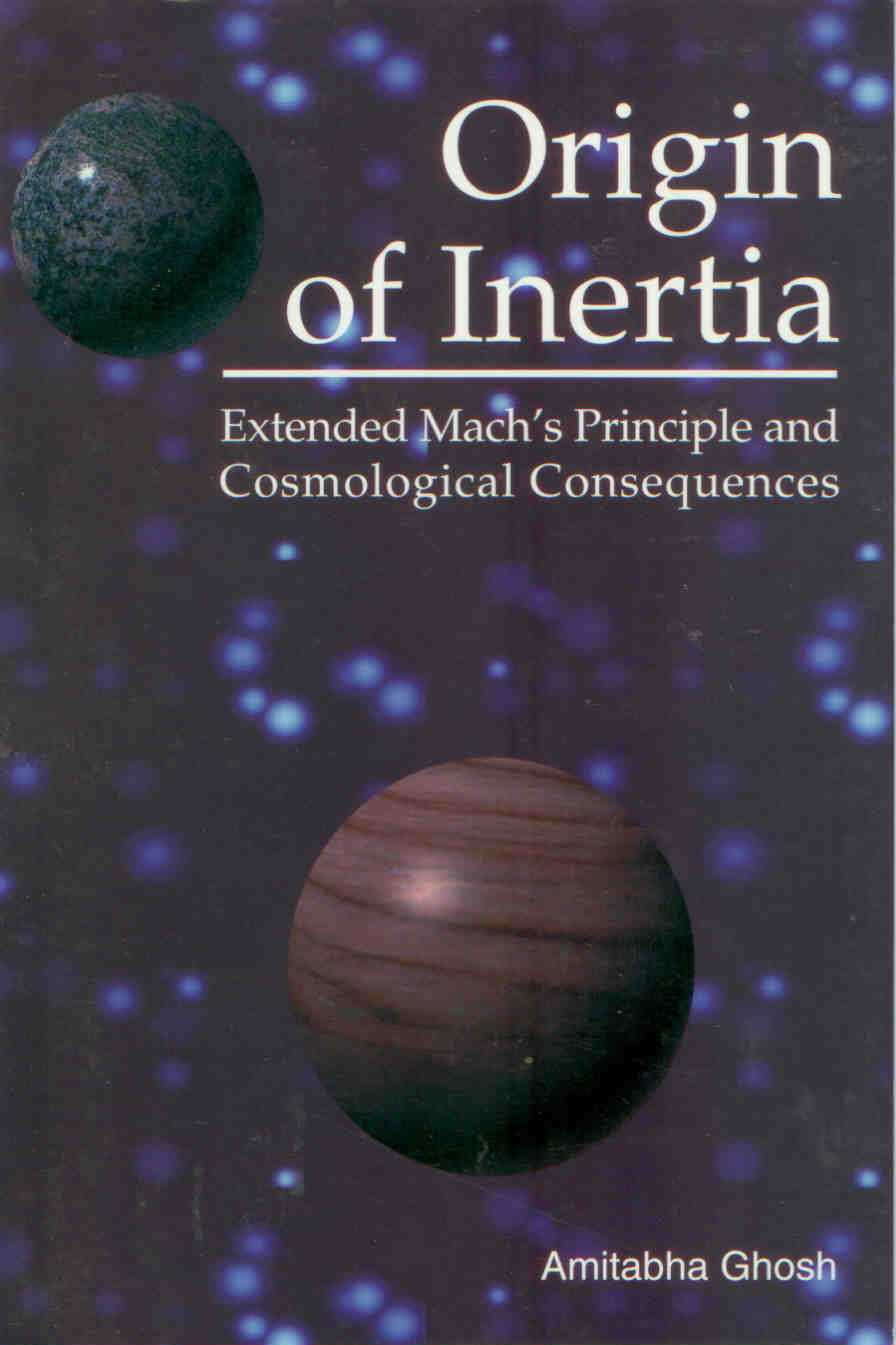Enter the content which will be displayed in sticky bar

# BookBuy Now
Pages: 147
Publisher: C. Roy Keys Inc. (Apeiron)
Year: 2000/2008
ISBN: 096836893X
ISBN: 978-0968368930

Origin of Inertia: Extended Machs Principle and Cosmological Consequences (Buy Now)

The primary theme of this monograph is a theory in which Newton's static gravitational interaction has been replaced by a new dynamic model. According to this theory the interactive gravitational force between two objects depends not only on the separation but also on the relative velocity and acceleration between the interacting bodies. In a sense it is an extension of Mach's Principle, and could be termed the Extended Mach's Principle. According to Mach's Principle a force acts on an accelerating object due to its interaction with the matter present in the rest of the universe. In the Extended Mach's Principle such an interactive force acts on a body due to its velocity (in the mean rest frame of the universe) also. This force has been termed the Cosmic Drag. The initial Chapters offer some general discussions and highlight certain interesting features of the fundamental problem of motion. It has been demonstrated that in a relational framework an absolute character can be assigned to displacement, and hence it is meaningful to talk about an absolute frame of reference in an infinite, non-evolving and quasistatic universe satisfying the Perfect Cosmological Principle. A brief account of the basic difficulties in the Newtonian formulation of mechanics is then presented, followed by a historical account of how the earlier researchers tried to resolve these issues through various suggestions for modifications in Newton's laws. The next chapter presents Sciama's attempt to quantify Mach's Principle and his model of acceleration dependent inertial induction. Next, this is extended to include a velocity-dependent inertial induction term. Though very small, the effect of the velocity dependent inertial induction term introduces some fundamental changes in the basic framework of mechanics, leading to a modified law of motion. The exact equivalence of gravitational and inertial masses emerges as a natural consequence of the dynamic gravitational interaction.